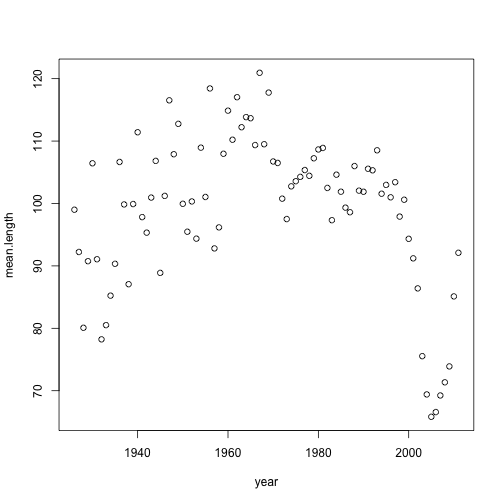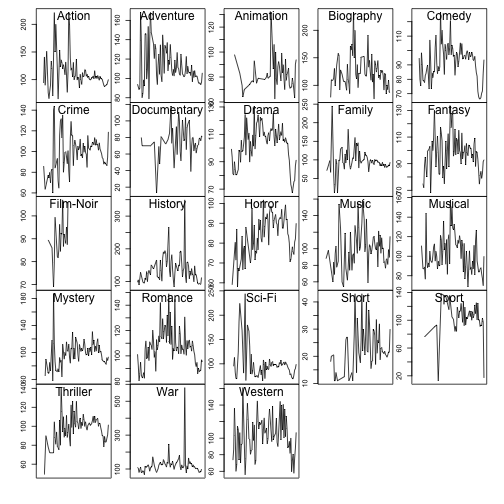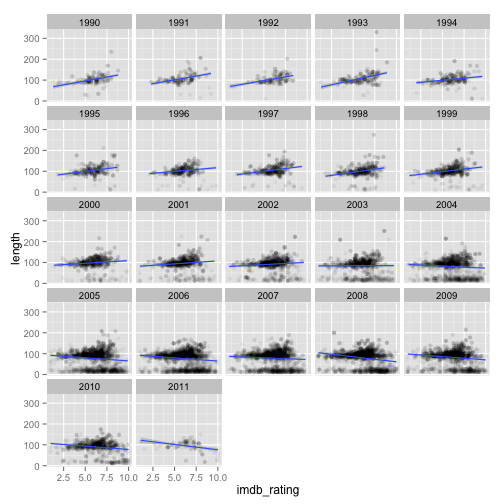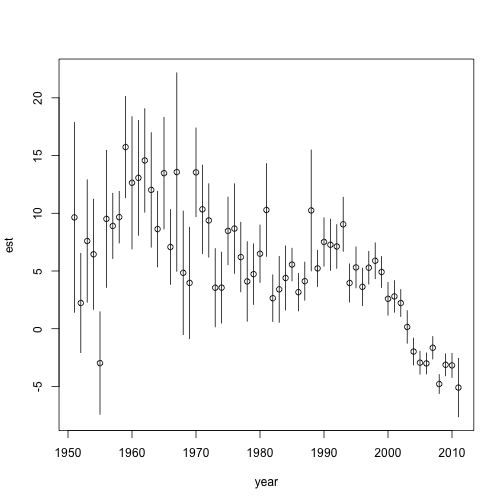# plyr examples

Let's load plyr and the data:

``````library(plyr)
``````

Let's start with a basic example. How does the mean movie length change through time?

``````x0 <- ddply(imdb, "year", summarize, mean.length = mean(length, na.rm = TRUE))
plot(x0)
``````We can also look at the mean length by genre and year. We can work group by multiple columns using the c(“column1”, “column2”) format.

``````x1 <- ddply(imdb, c("genre", "year"), summarize, mean.length = mean(length,
na.rm = TRUE))

par(mfrow = c(5, 5), mar = c(0, 1.2, 0, 1), oma = c(3, 3, 1, 1), cex = 0.6)
d_ply(x1, "genre", transform, {
plot(year, mean.length, type = "l", xaxt = "n", xlim = c(1920, 2011))
mtext(unique(genre), line = -1.5)
})
``````What if we wanted to detrend each time series with a linear model?

``````x2 <- ddply(subset(x1, !is.na(mean.length)), "genre", transform, residual = residuals(lm(mean.length ~
year)))
``````
``````##    genre year mean.length residual
## 1 Action 1926       94.00   -34.27
## 2 Action 1927      141.00    13.09
## 3 Action 1928       90.00   -37.55
## 4 Action 1930      151.00    24.17
## 5 Action 1932       76.67   -49.45
## 6 Action 1933       66.00   -59.75
``````

How about running a regression of movie length and rating for each year and storing the models?

``````x3 <- dlply(subset(imdb, year > 1950 & genre != "Short"), "year", function(x) lm(length ~
imdb_rating, data = x))
x3[]
``````
``````##
## Call:
## lm(formula = length ~ imdb_rating, data = x)
##
## Coefficients:
## (Intercept)  imdb_rating
##       32.21         9.64
``````

Now what if we wanted to extract the slopes and their confidence intervals from those models and return them in a data frame? You might want to step into the function with browser() to help write the code.

``````x4 <- ldply(x3, function(x) {
est <- coef(x)
ci <- confint(x)
ci.l <- ci[2, 1]
ci.u <- ci[2, 2]
data.frame(est, ci.l, ci.u)
})
library(ggplot2)
ggplot(subset(imdb, year >= 1990 & genre != "Short"), aes(imdb_rating, length)) +
geom_point(alpha = 0.05) + facet_wrap(~year) + stat_smooth(method = "lm")
````````````par(mfrow = c(1, 1))
with(x4, plot(year, est, ylim = c(min(ci.l), max(ci.u))))
d_ply(x4, "year", transform, segments(year, ci.l, year, ci.u))
``````